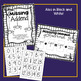# Missing Addend Fall Math Center FREEBIE!Subject
Resource Type
File Type

PDF

(2 MB|12 pages)
Product Rating
Standards
• Product Description
• StandardsNEW

Finding the missing addend is an excellent skill to practice and made super easy with this FREE math center! Simple to set up and ready to go for the October/Fall/Halloween season with this adorable theme.

To prepare center: Make copies of recording page per student. Laminate (if desired) mat and number cards and cut apart. Place in folder with recording pages at math centers.

To play game: Draw 3 cards and lay on mat. Find the Missing Addend and record on your answer page.

*PLEASE NOTE: This center can be found bundled in my Balancing Facts Math Centers! product.

Included:

• Large Balancing game
• Center Game cover pages
• Accountability sheets

All center materials in black and white, too!

This math center is designed to complement my MATH! Curriculum for first grade. You can find it HERE.

These are my Math Tubs:

M – Model It!

A – Add or Subtract It!

T – Think It!

H – Hone It!

IT – I’m Through!

You can read about how I set up my Math Centers HERE.

Thanks and happy teaching!!

Jessica

Like Tech and Teachability on Facebook

Fluently add and subtract within 20 using mental strategies. By end of Grade 2, know from memory all sums of two one-digit numbers.
Understand the meaning of the equal sign, and determine if equations involving addition and subtraction are true or false. For example, which of the following equations are true and which are false? 6 = 6, 7 = 8 - 1, 5 + 2 = 2 + 5, 4 + 1 = 5 + 2.
Add and subtract within 20, demonstrating fluency for addition and subtraction within 10. Use strategies such as counting on; making ten (e.g., 8 + 6 = 8 + 2 + 4 = 10 + 4 = 14); decomposing a number leading to a ten (e.g., 13 - 4 = 13 - 3 - 1 = 10 - 1 = 9); using the relationship between addition and subtraction (e.g., knowing that 8 + 4 = 12, one knows 12 - 8 = 4); and creating equivalent but easier or known sums (e.g., adding 6 + 7 by creating the known equivalent 6 + 6 + 1 = 12 + 1 = 13).
Relate counting to addition and subtraction (e.g., by counting on 2 to add 2).
Apply properties of operations as strategies to add and subtract. If 8 + 3 = 11 is known, then 3 + 8 = 11 is also known. (Commutative property of addition.) To add 2 + 6 + 4, the second two numbers can be added to make a ten, so 2 + 6 + 4 = 2 + 10 = 12. (Associative property of addition.)
Total Pages
12 pages
N/A
Teaching Duration
N/A
Report this Resource to TpT
Reported resources will be reviewed by our team. Report this resource to let us know if this resource violates TpT’s content guidelines.